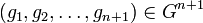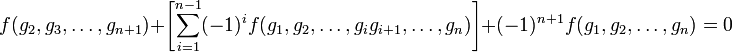# Cocycle for trivial group action

(diff) ← Older revision | Latest revision (diff) | Newer revision → (diff)

## Definition

Suppose$G$ is a group and$A$ is an abelian group.

### Definition in terms of cocycle for a group action

A$n$-cocycle for trivial group action is a$n$-cocycle for a group action of$G$ on$A$, where the action is trivial.

### Explicit definition

A$n$-cocycle for trivial group action of$G$ on$A$ is a function$f:G^n \to A$ satisfying the following for all$(g_1,g_2,\dots,g_{n+1}) \in G^{n+1}$:$\! f(g_2,g_3,\dots,g_{n+1}) + \left[ \sum_{i=1}^{n-1} (-1)^i f(g_1,g_2, \dots,g_ig_{i+1},\dots,g_n)\right] + (-1)^{n+1} f(g_1,g_2,\dots,g_n) = 0$

## Particular cases

Value of$n$ Condition for being a$n$-cocycle for trivial group action Further information
1$\! f(g_2) - f(g_1g_2) + f(g_1) = 0$, or$f(g_1g_2) = f(g_1) + f(g_2)$ It becomes a homomorphism of groups from$G$ to$A$, and hence, from the abelianization of$G$ to$A$
2$\! f(g_2,g_3) - f(g_1g_2,g_3) + f(g_1,g_2g_3) - f(g_1,g_2) = 0$, or$\! f(g_2,g_3) + f(g_1,g_2g_3) = f(g_1g_2,g_3) + f(g_1,g_2)$. 2-cocycle for trivial group action